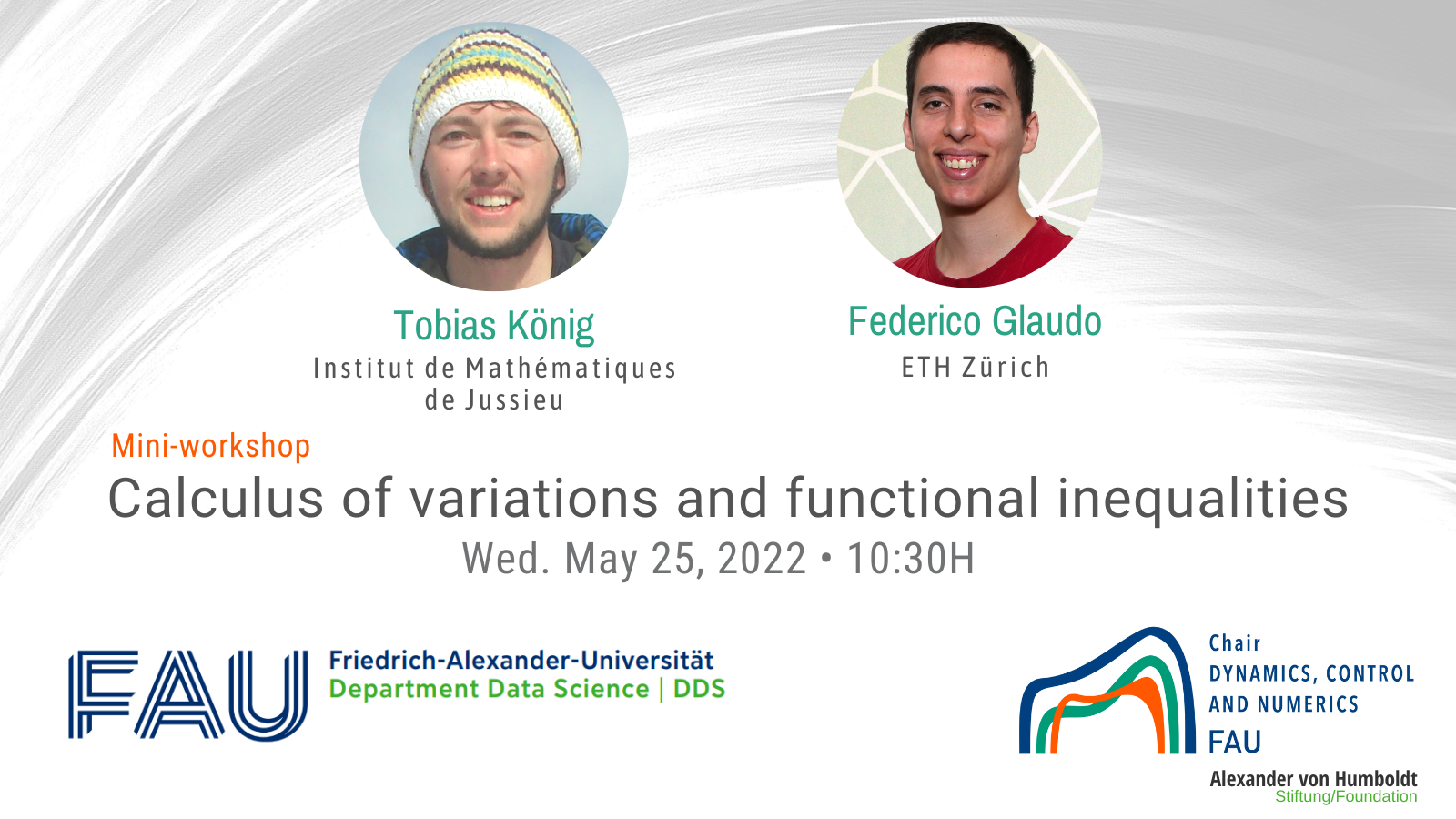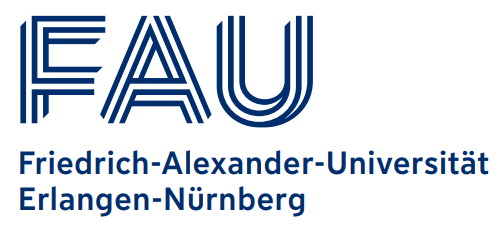# Mini-Workshop “Calculus of Variations and Functional Inequalities”

Date: Wed. May 25, 2022
Organized by: FAU DCN-AvH, Chair for Dynamics, Control and Numerics – Alexander von Humboldt Professorship at FAU Erlangen-Nürnberg (Germany) – Alexander von Humboldt Professorship at FAU Erlangen-Nürnberg (Germany)
Title: Mini-workshop “Calculus of Variations and Functional Inequalities”

WHERE?
This is a hybrid event (online & on-site)
Online: Join via Zoom meeting link
Meeting ID: 682 9425 7970 | PIN: 937764

On site: Felix Klein building. Room 03.323
Friedrich-Alexander-Universität Erlangen-Nürnberg.
Cauestrasse 11, 91058 – Erlangen, Bavaria (Germany)

10:30H
Tobias König, Institut de Mathématiques de Jussieu, Paris Rive Gauche
“The fractional Brezis–Nirenberg problem in low dimensions. Critical functions and blow-up asymptotics”

Abstract. The classical Brezis–Nirenberg problem asks for the existence, respectively non-existence, of positive solutions u to -\Delta u + a u = u^\frac{N+2}{N-2} on some domain \Omega \subset \R^N with zero Dirichlet boundary conditions, depending on the choice of a \in C(\overline{\Omega}). I will begin by discussing this problem, with emphasis on the special role of dimension N = 3 for its behavior.

I will then introduce the fractional version of the Brezis–Nirenberg problem involving the fractional Laplacian (-\Delta)^s with s \in (0,1) and the corresponding critical exponent \frac{N+2s}{N-2s}. It turns out that the problem now behaves specially in dimensions N \in (2s, 4s). For such dimensions, I will present some recent results joint with N. De Nitti (FAU DCN-AvH). Firstly, we characterize the functions a for which an energy-minimizing solution exists in terms of the Green’s function of (-\Delta)^s + a, thus extending a well-known result for s =1 due to Druet. Secondly, we give a precise description of the concentration behavior of minimizing solutions u_{\epsilon} associated to functions a_{\epsilon} tending to some critical a.

11:30H
Federico Glaudo, ETH Zürich
“On the sharp stability of critical points of the Sobolev inequality”

Abstract. The unique minimizers of the Sobolev inequality in R^n are known to be the Talenti bubbles, a two parameters (position and concentration) family of functions. As a consequence, the Talenti bubbles solve the associated Euler-Lagrange equation \Delta u + u^{2^*-1} = 0 in R^n.
If u : R^n \to R is a sum of “almost independent” bubbles, then u “almost solves” the Euler-Lagrange equation, that is |\Delta u + u^{2^*-1}|_{H^{-1}} \ll 1. M. Struwe proved the converse in the 80s, i.e., that if a function u satisfies |\Delta u + u^{2^*-1}|_{H^{-1}} \ll 1 then u is close in H^1 to a sum of almost independent bubbles.
With an application to the fast diffusion equation in mind, we will discuss the sharp quantitative stability of Struwe’s result. We will present various recent (sharp quantitative) estimates of the distance (in H^1) between u and the manifold of sum of Talenti bubbles with the quantity |\Delta u + u^{2^*-1}|_{H^{-1}}. The unexpected and novel feature is that the sharp exponent in these estimates depends on the dimension n.
This talk is based on a joint work with A. Figalli.

##### Previous FAU DCN-AvH Workshops:

If you like this, you don’t want to miss out our upcoming events!

### Date

Wed. May 25, 2022
Expired!

### Time

10:30 - 12:30